# 2. Assume two fair dice are rolled. Let X be the number showing on the first die and number showing on the second die. (a) Construct the matrix showing the joint probability mass function of the pair...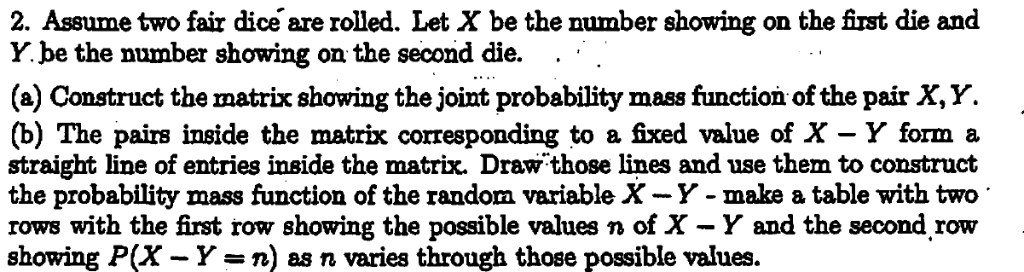2. Assume two fair dice are rolled. Let X be the number showing on the first die and number showing on the second die. (a) Construct the matrix showing the joint probability mass function of the pair X,Y. (b) The pairs inside the matrix corresponding to a fixed value of X - Y form a straight line of entries inside the matrix. Draw those lines and use them to construct the probability mass function of the random variable X-Y- make a table with two rows with the first row showing the possible values n of X - Y and the second row showing P(X - Y-n) as n varies through those possible values.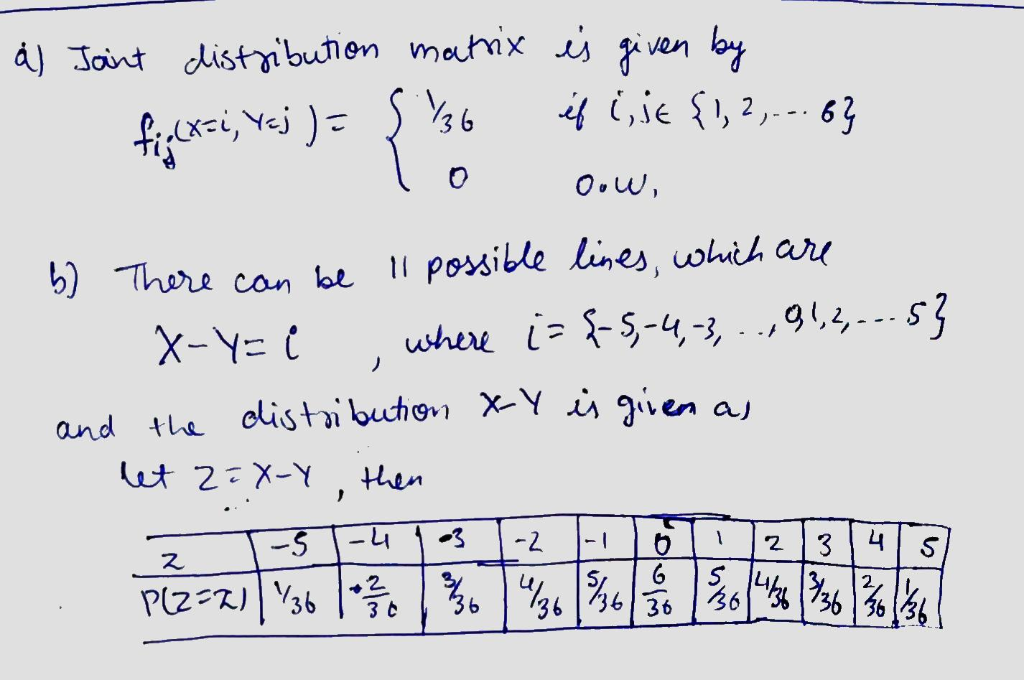##### Add Answer of: 2. Assume two fair dice are rolled. Let X be the number showing on the first die and number showing on the second die. (a) Construct the matrix showing the joint probability mass function of the pair...
Similar Homework Help Questions
• ### Two fair 6-sided dice are tossed. Let X denote the number appearing on the first die...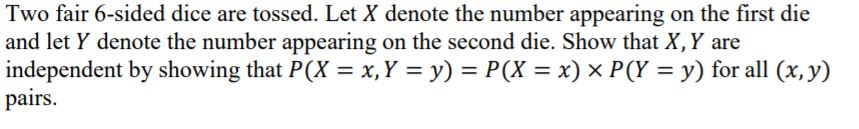Two fair 6-sided dice are tossed. Let X denote the number appearing on the first die and let y denote the number appearing on the second die. Show that X, Y are independent by showing that P(X = x, Y = y) = P(X = x) x P(Y = y) for all (x,y) pairs.

• ### 4. Two fair dice are rolled. Compute the probability that the number on the first is...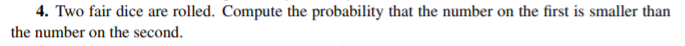4. Two fair dice are rolled. Compute the probability that the number on the first is smaller than the number on the second

• ### Two identical fair 6-sided dice are rolled simultaneously. Each die that shows a number less than...

Two identical fair 6-sided dice are rolled simultaneously. Each die that shows a number less than or equal to 4 is rolled once again. Let X be the number of dice that show a number less than or equal to 4 on the first roll, and let Y be the total number of dice that show a number greater than 4 at the end. (a) Find the joint PMF of X and Y . (Show your final answer in a...

• ### Two four-sided dice, one red and one white, will be rolled. List the possible values for the following random variable. Let Y = difference between the number on the red die and the number on the white...

Two four-sided dice, one red and one white, will be rolled. List the possible values for the following random variable. Let Y = difference between the number on the red die and the number on the white die (red-white). a. Draw the probability histogram for this random variable. b. What is the most likely value of Y? c. What is the probability that the difference on the dice is negative? Use proper notation throughout. Write your answer as a decimal.

• ### 3. A fair die is rolled twice. Let the first outcome be X and the second...3. A fair die is rolled twice. Let the first outcome be X and the second outcome be Y (a) (5 points) Calculate Cov(XY, X -Y) and simplify. (hint: What is Cov(aX+bY) in terms of Cov(X, Y)?) (b) (5 points) Are X Y and X -Y independent? Explain. (c) (5 points) Calculate the moment generating function Mx+y(t) of X+Y (the answer should be a function of t and can contain unsimplified sums)

• ### For the two six-sided dice case: Write out the six-by-six matrix showing all possible (36) combinations...

For the two six-sided dice case: Write out the six-by-six matrix showing all possible (36) combinations of outcomes. Draw a histogram of the probability of outcomes for the dice totals. Explain the shape of the histogram. Draw a Venn diagram for the 36 dice roll combinations. Define a set "A" as all the combinations that total seven; define set "B" as all the combinations that have one die roll (either die 1 or 2) equal to 2. Indicate the sets...

• ### Let X and Y equal the outcomes when two fair six-sided dice are rolled

Let X and Y equal the outcomes when two fair six-sided dice are rolled. Let W = X + Y. Assuming independence, find the p.m.f. of W when (a) The first die has three faces numbered 0 and three faces numbered 2, and the second die has its faces numbered 0, 1, 4, 5, 8, and 9. (b) The faces on the first die are numbered 0, 1, 2, 3, 4, and 5, and the faces on the second die...

• ### Please answer the question clearly 6. Suppose that we roll a pair of balanced dice. Let...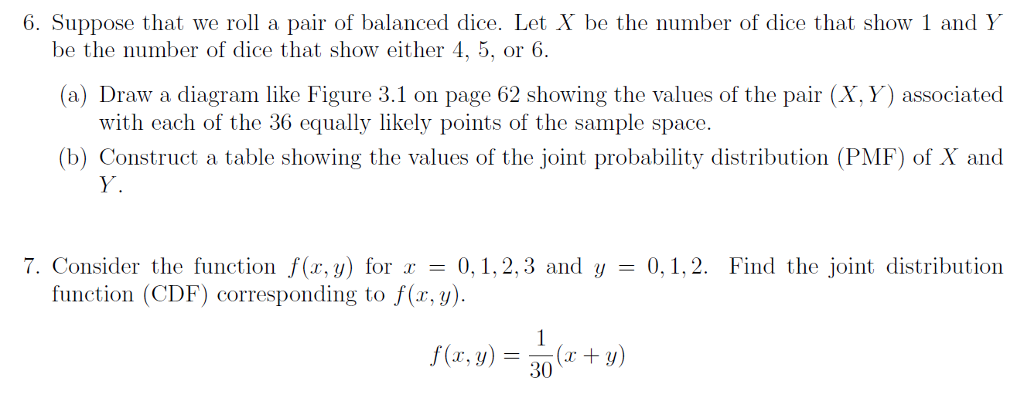Please answer the question clearly 6. Suppose that we roll a pair of balanced dice. Let X be the number of dice that show 1 and Y be the number of dice that show either 4, 5, or 6. (a) Draw a diagram like Figure 3.1 on page 62 showing the values of the pair (X, Y) associated with each of the 36 equally likely points of the sample space. (b) Construct a table showing the values of the joint...

• ### Probability mass function of (X, Y)

Two dice are tossed. Let X be the smaller number of points. Let Y be the larger number of points. If both dice show the same number, say, z points, then X=Y=z.a) Find the joint probability mass function of (X,Y).b) Are X and Y independent? Explain.c) Find the distribution (probability mass function) of X.d) If X=2, what is the probability that Y=5?

• ### Roll two fair dice

Roll two fair dice. what is the probability that you get a 2 and a 5 without regard to which is on which die? What is the probability of at least one 2 or one 5? What is the probability of a sum of 7? "what is the probability that you get a 2 and a 5 without regard to which is on which die? " 1/6* 1/6* 2 = 1/18 There are two possibilities both with probability 1/6 *...

Need Online Homework Help?